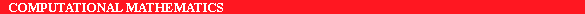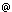ERCIM News No.22 - July 1995 - SZTAKI

# Free Boundary Problems in Reaction-Diffusion Systems and in Turbulence Modelling

by Robert Kersner

Free boundary problems (FBP) constitute a mathematical research topic characterized by the occurrence of frontiers whose location are a priori unknown. These problems are currently being studied by the Laboratory of Dynamic and Discrete Structures of SZTAKI. The objective of the research is to promote the mathematical and numerical treatment of FBPs starting from modelling and including all relevant theoretical studies.

Free boundaries separate space-time regions with different properties. As an example, we can consider the solidification of water where the location of the boundary between water and ice is not fixed and changes during the process. Free boundaries occur naturally in the mathematical formulation of a great variety of scientific and technological processes, e.g. in material processing (steel casting, crystal and dendritic growth, etc.), in biology (population dynamics, growth of bacteria), in combustion theory, in reaction-diffusion problems, in electrochemistry or in fluid flow-through porous media.

These types of FBPs have received little attention from mathematicians until recently when, in the sixties-seventies, the modern approach to the theory of nonlinear partial differential equations brought new insight and new methods to their mathematical study. Over the past two decades, the great development in this sector has established it as an important interdisciplinary area covering topics in applied mathematical modelling and in mathematics, such as partial and ordinary differential equations, numerical and functional analysis, calculus of variations and optimal control, differential geometry. The study of such problems raises profound issues in nonlinear analysis, as well as causing new and difficult questions to emerge in numerical analysis and scientific computation.

We are now studying these problems in the Laboratory of Dynamic and Discrete Structures of SZTAKI. A major project is focussed on the study of the propagation of gas or liquids in porous media in the presence of evaporation, sources and/or absorbers. This problem is associated with a class of degenerate (non uniformly parabolic) nonlinear partial differential equations, the coefficients of which are functions of the unknown density. The free boundary is the boundary of the saturated zone. The same model describes the nonlinear heat conduction with convection and absorption with temperature dependent heat conduction coefficient, intensity of convection and absorption. As a rule, the solution (density, temperature) is not smooth on the free boundary, which makes the identification of the free boundaries very complicated.

There are many technologically significant problems where turbulence modelling can be used to generate useful engineering solutions. One of the most widely used models is the k-epsilon turbulence model which can be derived from Navier-Stokes system with the help of the Kolmogorov and Prandtl hypothesis. In the simplest situation, the model consists of two strongly coupled singular parabolic equations, one for k (the turbulence energy) and the other for epsilon (dissipation rate). The free boundary, dividing the domains where k is positive and k is zero, is in fact the geometric boundary of the turbulent domain. The free boundary depends on certain system parameters in a very surprising way. Our results produce a method to estimate these parameters, which otherwise could only be estimated using expensive experiments. We have found several new, explicit solutions which are four-parameter families and show, for instance, the self-similar behaviour of the process in the physical range of system parameters.

E-mail: h1019kerella.hu